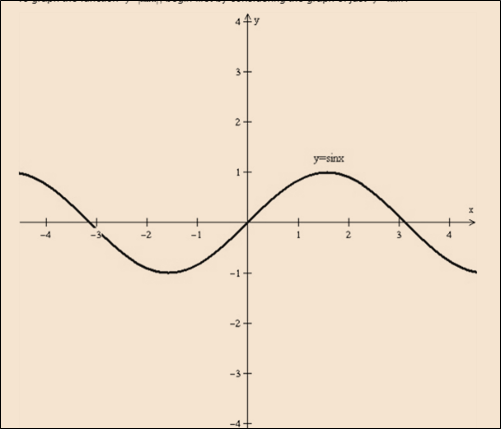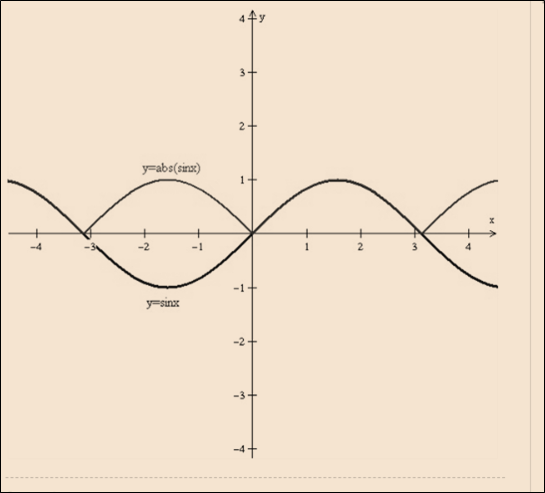# To graph: The following function with the help of the transformation: y = | sin x |### Single Variable Calculus: Concepts...

4th Edition
James Stewart
Publisher: Cengage Learning
ISBN: 9781337687805### Single Variable Calculus: Concepts...

4th Edition
James Stewart
Publisher: Cengage Learning
ISBN: 9781337687805

#### Solutions

Chapter C, Problem 40E
To determine

## To graph: The following function with the help of the transformation:   y=|sinx|

Expert Solution

### Explanation of Solution

Given information:

y=|sinx|

Graph:Graph 1: y=sinxGraph 2: y=|sinx|

Interpretation:

Need to draw a first graph of the function y=cosx as shown by the graph 1. Except positive output, need to recall all possible values of function or sine to take same domain as original.

For example:

One cycle of sine where x in interval [02π] , in this case the function is already positive. For the remainder of cycle y should be negative. So, here graph changes from original.

The same shape is observed for the second half of the graph, except its flipped shape across x axis which results that y values are positive.

### Have a homework question?

Subscribe to bartleby learn! Ask subject matter experts 30 homework questions each month. Plus, you’ll have access to millions of step-by-step textbook answers!